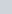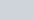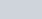A person can row a boat at the rate of 5 km/hour
Question:

A person can row a boat at the rate of 5 km/hour in still water. He takes thrice as much time in going 40 km upstream as in going 40 km downstream. Find the speed of the stream.

Solution:

Given that,

Speed of boat in still water = 5 km/hour.

Distance covered by boat = 40 km

Now we are assuming that the speed of the stream is, then

Speed of the boat rowing upstream =, and

Speed of the boat rowing downstream =According to the given condition, time taken to coverin upstream is three times the time taken to coverin downstream, therefore

$\frac{40}{5-v}=3 \times \frac{40}{5+v}$

$\Rightarrow 5+v=15-3 v$

$\Rightarrow \quad 4 v=10$

$\Rightarrow \quad v=2.5$

Hence the speed of stream is 2.5 km/hour.